# WPF怎么做新手引导界面？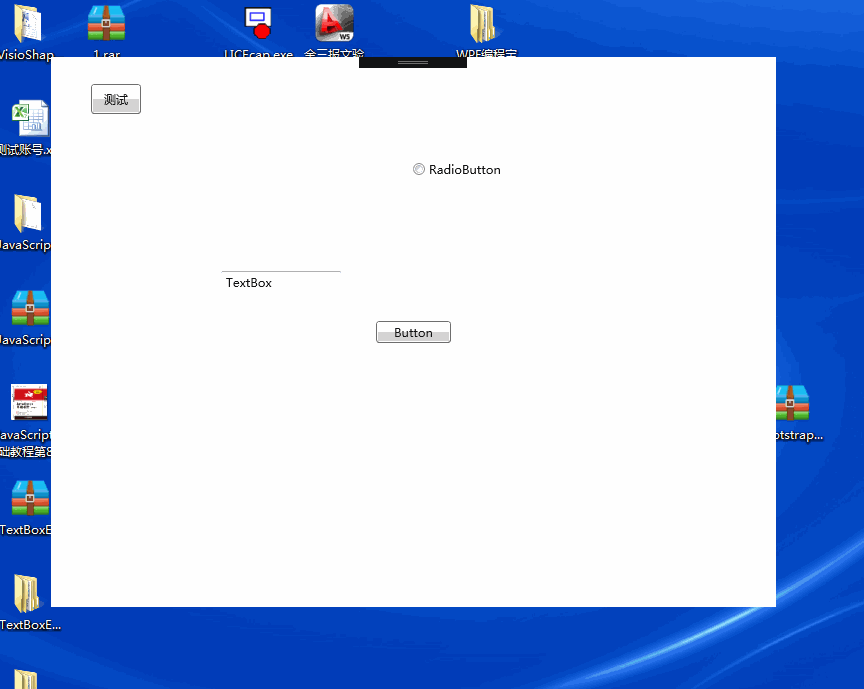## 一、遮罩窗体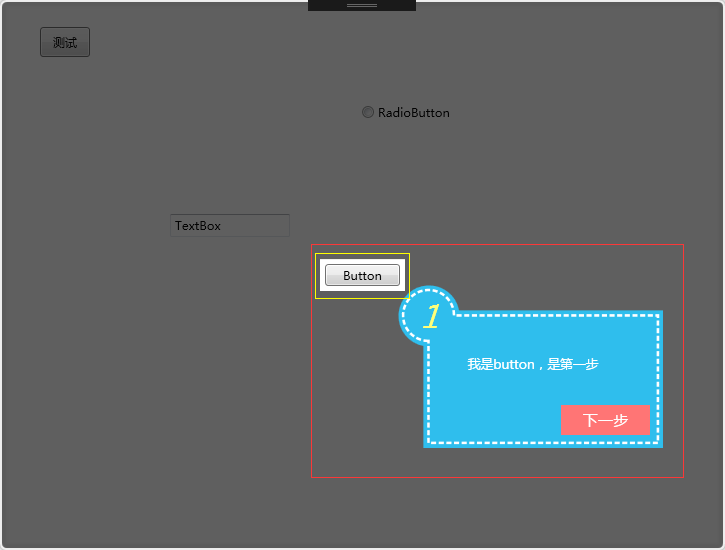``````<Window x:Class="SimpleGuide.GuideWin" xmlns="http://schemas.microsoft.com/winfx/2006/xaml/presentation" xmlns:x="http://schemas.microsoft.com/winfx/2006/xaml" xmlns:d="http://schemas.microsoft.com/expression/blend/2008" xmlns:mc="http://schemas.openxmlformats.org/markup-compatibility/2006" xmlns:local="clr-namespace:SimpleGuide" mc:Ignorable="d" Title="GuideWin" WindowStyle="None" AllowsTransparency="True" x:Name="gw" Background="#01FFFFFF" ShowInTaskbar="False">
<Grid>
<Border x:Name="bor" BorderBrush="White" BorderThickness="2" CornerRadius="5" Opacity="0.8">
<Border.Effect>
</Border.Effect>
<Border Background="Black" Opacity="0.5" Margin="0" CornerRadius="5" />
</Border>
<Canvas x:Name="can"></Canvas>
</Grid>
</Window>
``````

## 二、显示要操作的控件

``````Point point = fe.TransformToAncestor(Window.GetWindow(fe)).Transform(new Point(0, 0));
``````

``````RectangleGeometry rg1 = new RectangleGeometry();
rg1.Rect = new Rect(point.X - 5, point.Y - 5, fe.ActualWidth + 10, fe.ActualHeight + 10);
borGeometry = Geometry.Combine(borGeometry, rg1, GeometryCombineMode.Exclude, null);
``````

## 三、绘制一个指引的UC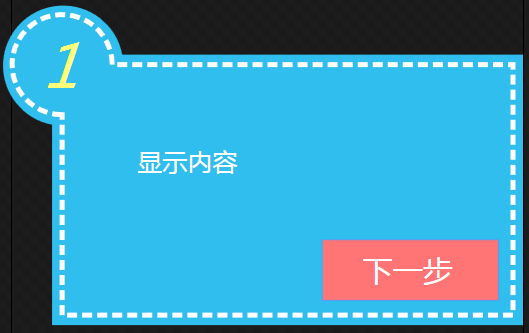``````<Path Fill="#FF2FBEED">
<Path.Data>
<GeometryGroup>
<PathGeometry Figures="M 8,22 A 12,12 0 1 1 22,8 L 102 8 L 102 62 L 8 62 Z" />
</GeometryGroup>
</Path.Data>
</Path>
<Path StrokeThickness="1" Stroke="White" StrokeDashArray="2,1" Fill="#FF2FBEED">
<Path.Data>
<GeometryGroup>
<PathGeometry Figures="M 10,20 A 10,10 0 1 1 20,10 L 100 10 L 100 60 L 10 60 Z" />
</GeometryGroup>
</Path.Data>
</Path>
``````

``````public HintUC(string xh, string content, Visibility vis = Visibility.Visible, int width = 260, int height = 160)
{
InitializeComponent();
this.Width = width;
this.Height = height;
this.tb_nr.Width = width / 4;

this.tb_xh.Text = xh;
this.tb_nr.Text = content;
this.btn_next.Visibility = vis;
}
``````

## 四、下一步的触发

``````private void show(int xh, FrameworkElement fe, string con, Visibility vis = Visibility.Visible)
{
Point point = fe.TransformToAncestor(Window.GetWindow(fe)).Transform(new Point(0, 0));//获取控件坐标点

RectangleGeometry rg = new RectangleGeometry();
rg.Rect = new Rect(0, 0, this.Width, this.Height);
borGeometry = Geometry.Combine(borGeometry, rg, GeometryCombineMode.Union, null);
bor.Clip = borGeometry;

RectangleGeometry rg1 = new RectangleGeometry();
rg1.Rect = new Rect(point.X - 5, point.Y - 5, fe.ActualWidth + 10, fe.ActualHeight + 10);
borGeometry = Geometry.Combine(borGeometry, rg1, GeometryCombineMode.Exclude, null);

bor.Clip = borGeometry;

HintUC hit = new HintUC(xh.ToString(), con, vis);
Canvas.SetLeft(hit, point.X + fe.ActualWidth + 3);
Canvas.SetTop(hit, point.Y + fe.ActualHeight + 3);
hit.nextHintEvent -= Hit_nextHintEvent;
hit.nextHintEvent += Hit_nextHintEvent;

index++;
}
``````
``````private void Hit_nextHintEvent()
{
if (list[index - 1] != null)
{
can.Children.Clear();
}
if (index == list.Count - 1)
show(index + 1, list[index].Uc, list[index].Content, Visibility.Collapsed);
else
show(index + 1, list[index].Uc, list[index].Content);
}
``````

## 五、扩展部分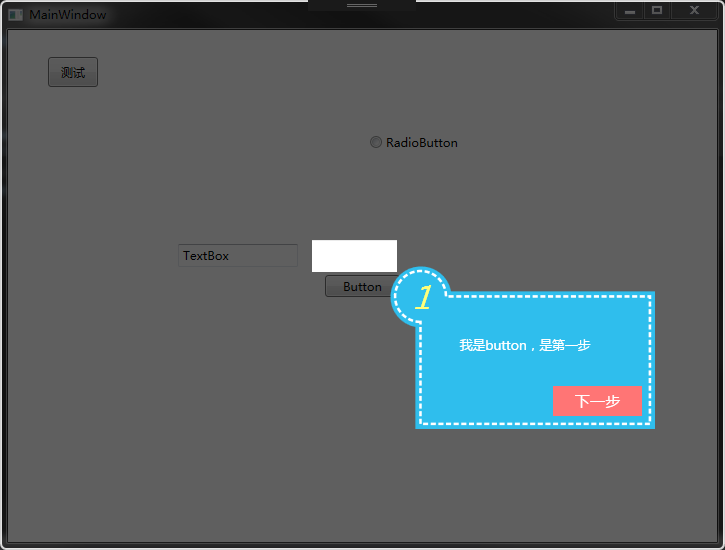## 站长使用体验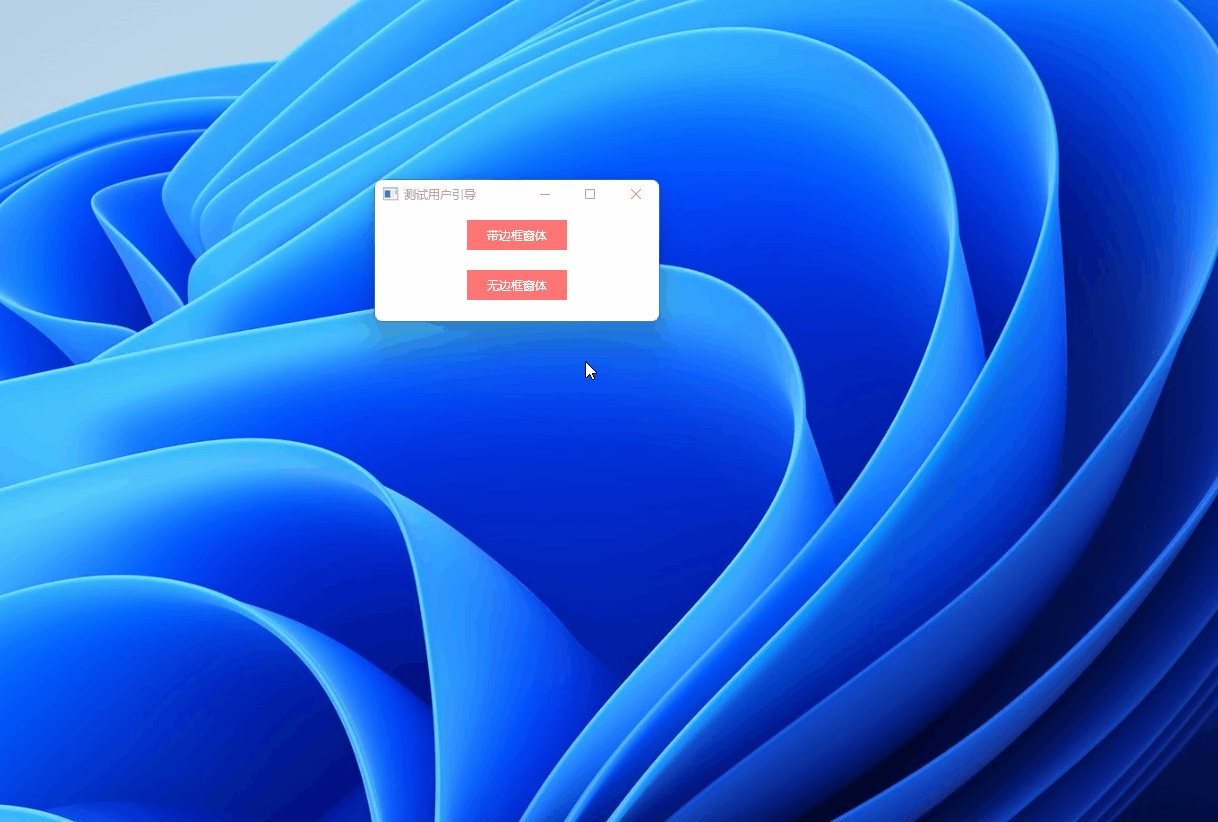## 文章评论

• 个人微信扫码工作时间

周一至周日 9:00-21:00

• 如果你觉得本站很棒，可以通过扫码支付打赏哦！

•微信收款码
•支付宝收款码
•QQ收款码